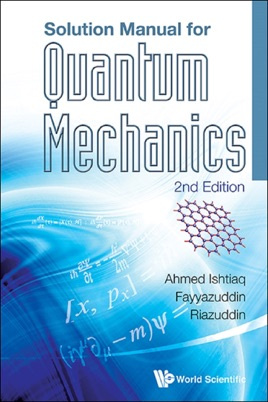• \$52.99

## Publisher Description

This is the solution manual for Riazuddin's and Fayyazuddin's Quantum Mechanics (2nd edition). The questions in the original book were selected with a view to illustrate the physical concepts and use of mathematical techniques which show their universality in tackling various problems of different physical origins. This solution manual contains the text and complete solution of every problem in the original book. This book will be a useful reference for students looking to master the concepts introduced in Quantum Mechanics (2nd edition).Contents:

Breakdown of Classical Concepts
Quantum Mechanical Concepts
Basic Postulates of Quantum Mechanics
Solution of Problems in Quantum Mechanics
Simple Harmonic Oscillator
Angular Momentum
Motion in Centrally Symmetric Field
Collision Theory
Operators
Heisenberg Equation of Motion, Invariance Principle and Path Integral
Angular Momentum and Spin
Time Independent Perturbation Theory
Time Dependent Perturbation Theory
Statistics and the Exclusion Principle
Two State Systems
Quantum Computation
Perturbation Induced by Electromagnetic Field
Formal Theory of Scattering
S-Matrix and Invariance Principles
Relativistic Quantum Mechanics: Dirac Equation
Dirac Equation in (1+2) Dimensions: Application to Graphene

Readership: Students and teachers of quantum mechanics.
Key Features:

It's a solution manual
Pretty self-explanatory